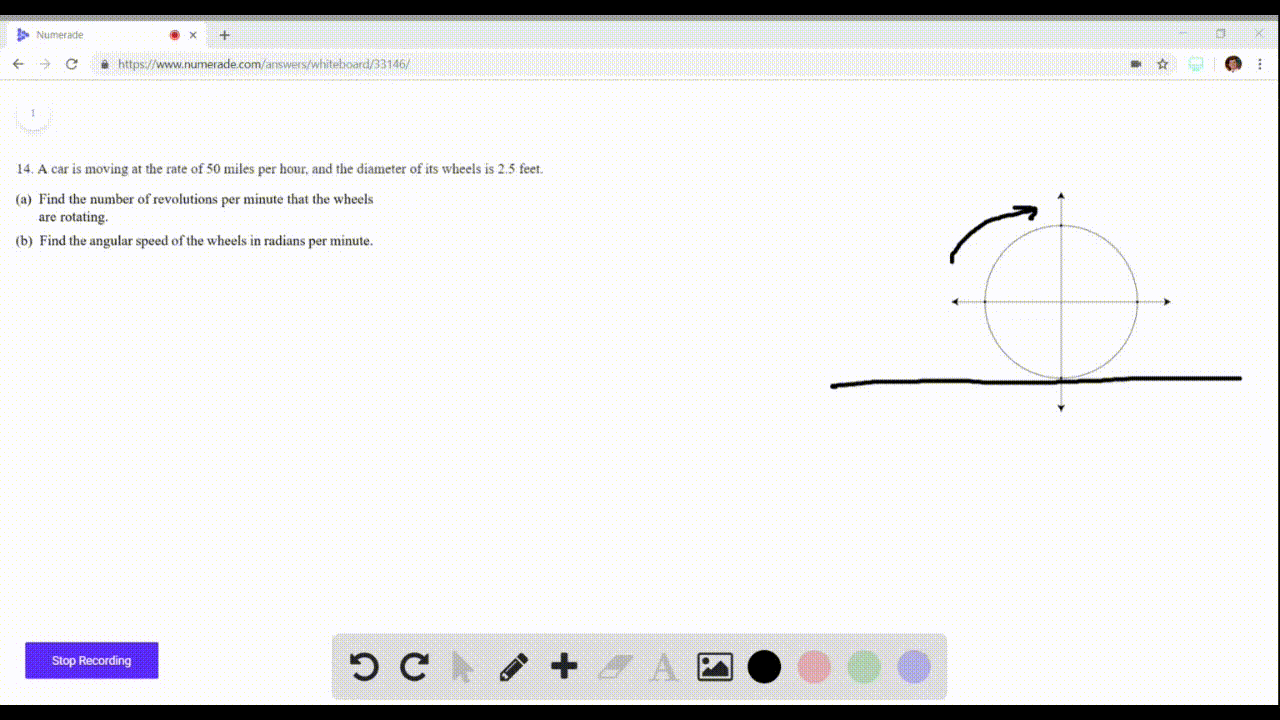🎉 The Study-to-Win Winning Ticket number has been announced! Go to your Tickets dashboard to see if you won! 🎉View Winning Ticket### Angular Speed A car is moving at the rate of 50 m…

04:06University of California, Berkeley
Problem 13

# Completing a Table Let $r$ represent the radius of a circle, $\theta$ the central angle (measured in radians), and s the length of the arc subtended by the angle. Use the relationship $s=r \theta$ to complete the table.

## Discussion

You must be signed in to discuss.

## Video Transcript

Okay, so we are asked to use the relationship. People are time data defying the missing piece. So for the first part, we have our eight feet. Actually, For the first part, we're trying to find data, so it's all through data that is equal to over our is 12 are eight. We get 1.5. Okay. For a second box we have our is 15. Data is 1.6 or something. So that is equal to our time. Does that 15 times. One point, Which gives me what for? Okay, now, for the third box, we're still finding s. So we use the same equations. That's s difficult to 85 times reply over four, which gives me 255 No. Okay. For the fourth and fifth box we're gonna solve for all right, So are is equal to s over there. You cannot open for our Arctic s, which is 96 over four. So we get 21 and then are equal to benefit book, which is a 642 over data, which is two pi over four to fire three. And that gives me 1 to 6, 93 pie. We have 12693 pipes. There we have it.